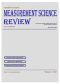MEASUREMENT SCIENCE REVIEW                Volume 2,  Section 1                    Main Page

 PAPER SECTION 1 Download and print the front cover  ->>Theoretical Problems of Measurement

1-7

Wimmer G., Witkovsky V.:
Proper Rounding of the Measurement Results under the Assumption of Uniform Distribution

Abstract:  In the paper we describe a new application of the ε-proper rounding method to measured values and their uncertainties and describe exactly the probability properties of the rounded measured values and their  ” ε-proper confidence intervals”. We propose general rules for proper rounding of the measurement results under uniform distribution assumptions.

9-20

Palenčár R., Wimmer G., Halaj M.:

Abstract: The paper shows a calibration model where a set of weights is calibrated in terms of the mass of a single reference weight. It is proved that under satisfying a simple condition the uncertainty of the reference mass value does not influence the mass values of the unknown weights, i.e. the calibration procedure is equivalent to a weighted least squares analysis. A simplified example demonstrates this calibration process.

21-31 Wimmer G., Witkovsky V., Duby T.:

Abstract:  In this paper we propose general rules for proper rounding of the measurement results based on the method called  ” ε - properly rounding ”  under triangular distribution assumptions.

33-42

Nalepa J.:

Abstract: The results of the simulation study on the differential algorithm of the correction of the dynamic error by the „blind” method are presented in the paper. The study was carried out for measurement channels modelled as first- and second-order systems. The results confirmed the suitability of computer simulation for determining the condition for the practical applicability of the discussed correction method.